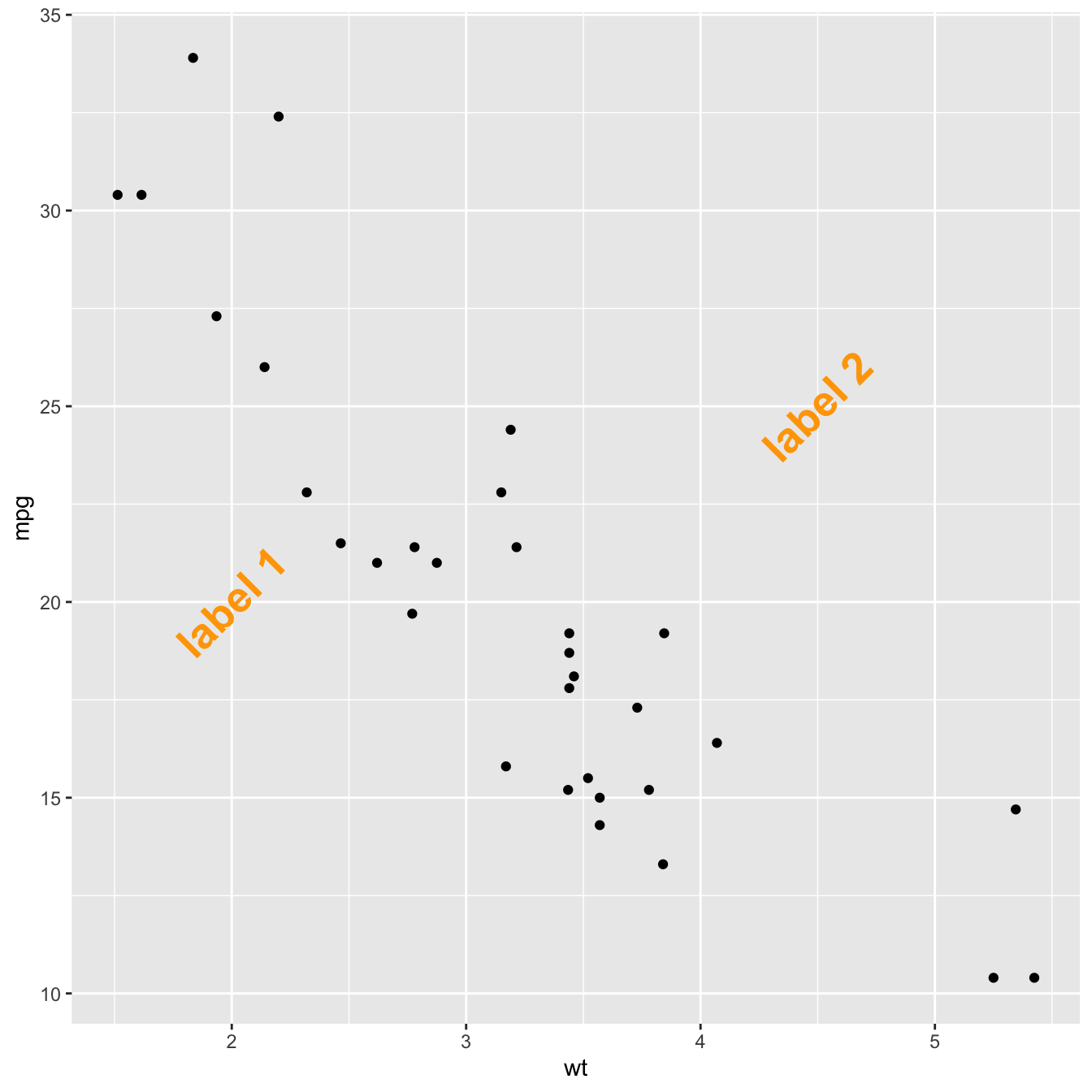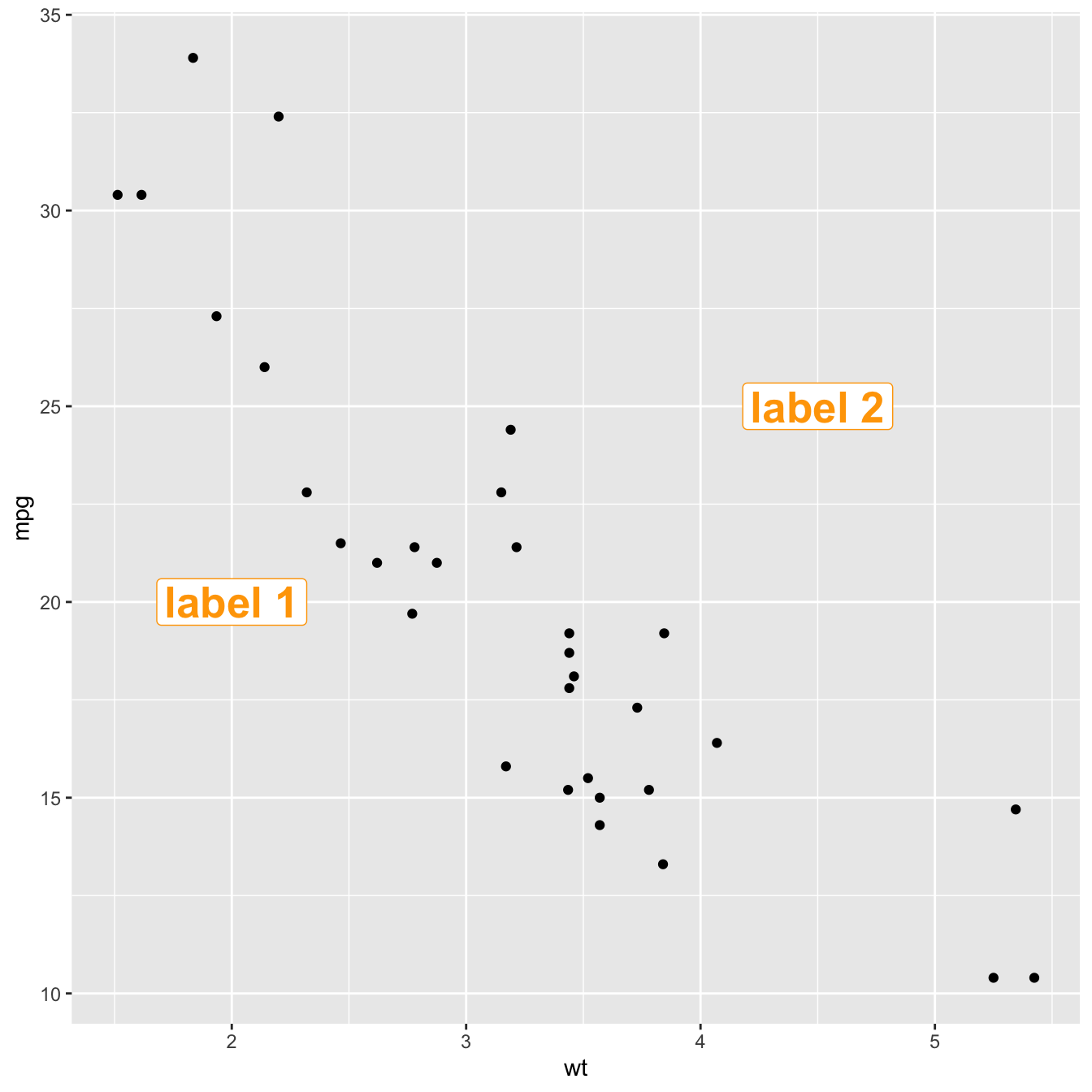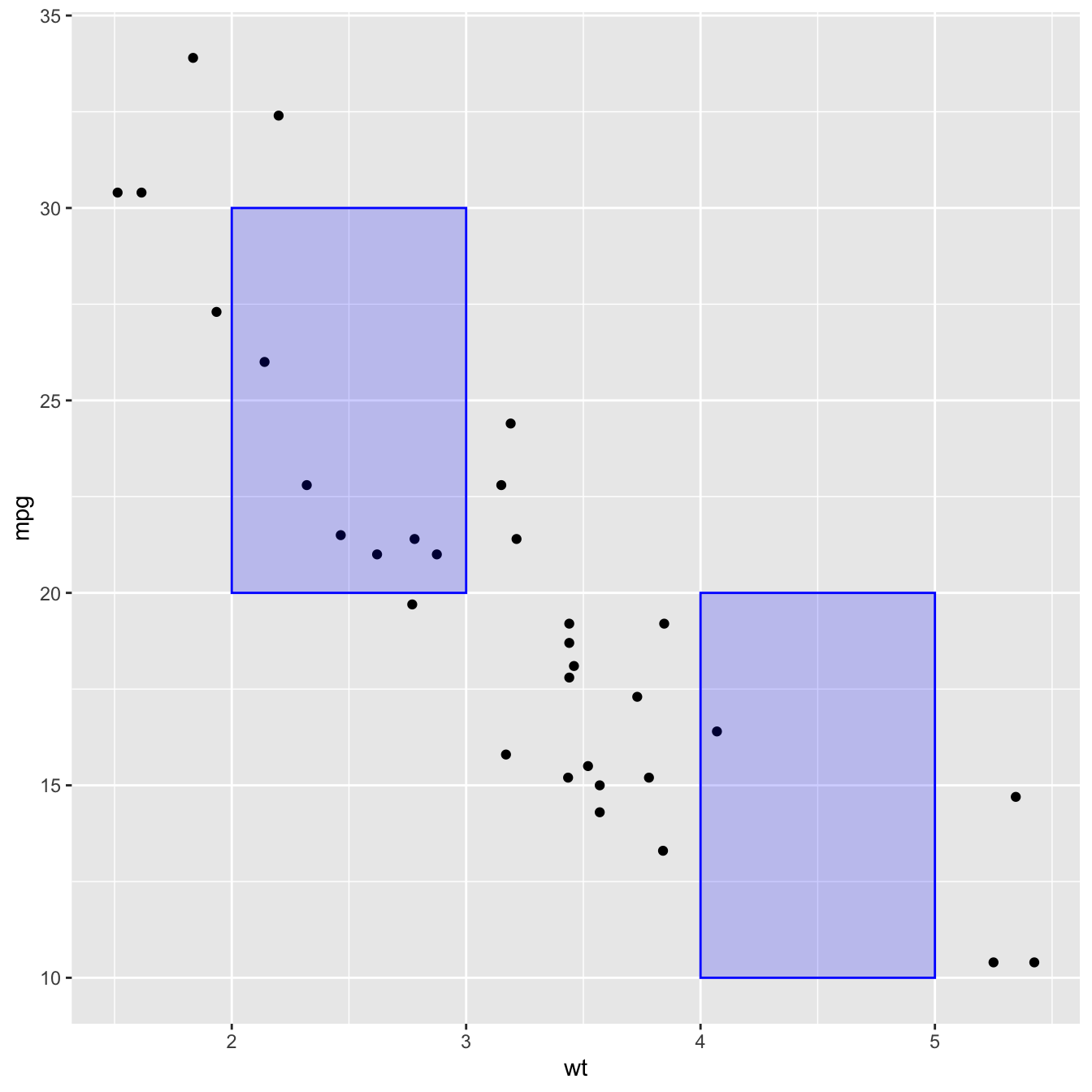# How to annotate a plot in ggplot2

Once your chart is done, annotating it is a crucial step to make it more insightful. This post will guide you through the best practices using `R` and `ggplot2`.

# Adding text with `geom_text()` or `geom_label()`Text is the most common kind of annotation. It allows to give more information on the most important part of the chart.

Using `ggplot2`, 2 main functions are available for that kind of annotation:

• `geom_text` to add a simple piece of text
• `geom_label` to add a label: framed text

Note that the `annotate()` function is a good alternative that can reduces the code length for simple cases.

``````# library
library(ggplot2)

# basic graph
p <- ggplot(mtcars, aes(x = wt, y = mpg)) +
geom_point()

# a data frame with all the annotation info
annotation <- data.frame(
x = c(2,4.5),
y = c(20,25),
label = c("label 1", "label 2")
)

p + geom_text(data=annotation, aes( x=x, y=y, label=label),                 ,
color="orange",
size=7 , angle=45, fontface="bold" )

# Note: possible to shorten with annotate:
# p +
#  annotate("text", x = c(2,4.5), y = c(20,25),
#           label = c("label 1", "label 2") , color="orange",
#           size=7 , angle=45, fontface="bold")``````
``````# Right chart: using labels
p + geom_label(data=annotation, aes( x=x, y=y, label=label),                 ,
color="orange",
size=7 , angle=45, fontface="bold" )``````

# Add shapes with `annotate()`The `annotate()` function allows to add all kind of shape on a `ggplot2` chart. The first argument will control what kind is used: `rect` or `segment` for rectangle, segment or arrow.

``````# Add rectangles
p + annotate("rect", xmin=c(2,4), xmax=c(3,5), ymin=c(20,10) , ymax=c(30,20), alpha=0.2, color="blue", fill="blue")``````
``````# Add segments
p + annotate("segment", x = 1, xend = 3, y = 25, yend = 15, colour = "purple", size=3, alpha=0.6)``````
``````# Add arrow
p + annotate("segment", x = 2, xend = 4, y = 15, yend = 25, colour = "pink", size=3, alpha=0.6, arrow=arrow())``````

# Add ablines with `geom_hline()` and `geom_vline()`

An abline is a segment that goes from one chart extremity to the other. `ggplot2` offers the `geom_hline()` and `geom_vline()` functions that are dedicated to it.``````p +
# horizontal
geom_hline(yintercept=25, color="orange", size=1) +
# vertical
geom_vline(xintercept=3, color="orange", size=1)``````

# Add a point and a range with.. `pointrange()`

Last kind of annotation, add a dot and a segment directly with `pointrange()`.``````# Add point and range
p + annotate("pointrange", x = 3.5, y = 20, ymin = 12, ymax = 28,colour = "orange", size = 1.5, alpha=0.4)``````

Related chart types

## Contact

This document is a work by Yan Holtz. Any feedback is highly encouraged. You can fill an issue on Github, drop me a message on Twitter, or send an email pasting yan.holtz.data with gmail.com.